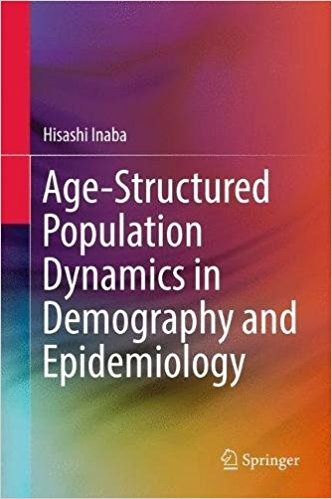Refer a friend and get % off! They'll get % off too.# Age-Structured Population Dynamics in Demography and Epidemiology (High Quality PDF)

This book is the first one in which basic demographic models are rigorously formulated by using modern age-structured population dynamics, extended to study real-world population problems. Age structure is a crucial factor in understanding population phenomena, and the essential ideas in demography and epidemiology cannot be understood without mathematical formulation; therefore, this book gives readers a robust mathematical introduction to human population studies. In the first part of the volume, classical demographic models such as the stable population model and its linear extensions, density-dependent nonlinear models, and pair-formation models are formulated by the McKendrick partial differential equation and are analyzed from a dynamical system point of view. In the second part, mathematical models for infectious diseases spreading at the population level are examined by using nonlinear differential equations and a renewal equation. Since an epidemic can be seen as a nonlinear renewal process of an infected population, this book will provide a natural unification point of view for demography and epidemiology. The well-known epidemic threshold principle is formulated by the basic reproduction number, which is also a most important key index in demography. The author develops a universal theory of the basic reproduction number in heterogeneous environments. By introducing the host age structure, epidemic models are developed into more realistic demographic formulations, which are essentially needed to attack urgent epidemiological control problems in the real world.

You will get a PDF (6MB) file

\$ 59.99

\$ 59.99

Discount has been applied.

or